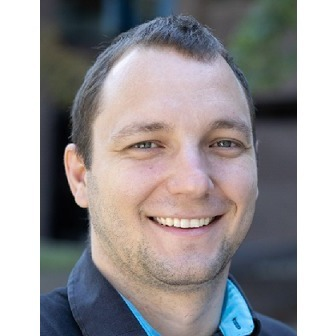CppCon 2021 has ended
Back To Schedule
Tuesday, October 26 • 7:45am - 8:45am

#### Log in to save this to your schedule, view media, leave feedback and see who's attending!

Feedback form is now closed.
Mathematical derivatives are vital components of many computing algorithms including: neural networks, numerical optimization, Bayesian inference, nonlinear equation solvers, physics simulations, sensitivity analysis, and nonlinear inverse problems. Derivatives track the rate of change of an output parameter with respect to an input parameter, such as how much reducing an individuals’ carbon footprint will impact the Earth’s temperature. Derivatives (and generalizations such as gradients, jacobians, hessians, etc) allow us to explore the properties of a function and better describe the underlying process as a whole. In recent years, the use of gradient-based optimizations such as training neural networks have become widespread, leading to many languages making differentiation a first-class citizen.

Derivatives can be computed numerically, but unfortunately the accumulation of floating-point errors and high-computational complexity presents several challenges. These problems become worse with higher order derivatives and more parameters to differentiate.

Many derivative-based algorithms require gradients, or the computation of the derivative of an output parameter with respect to many input parameters. As such, developing techniques for computing gradients that are scalable in the number of input parameters is crucial for the performance of such algorithms. This paper describes a broad set of domains where scalable derivative computations are essential. We make an overview of the major techniques in computing derivatives, and finally, we introduce the flagman of computational differential calculus -- algorithmic (also known as automatic) differentiation (AD). AD makes clever use of the ‘nice’ mathematical properties of the chained rule and generative programming to solve the scalability issues by inverting the dependence on the number of input variables to the number of output variables. AD provides mechanisms to augment the regular function computation with instructions calculating its derivatives.

Differentiable programming is a programming paradigm in which the programs can be differentiated throughout, usually via automatic differentiation. This talk introduces the differentiable programming paradigm in terms of C++. It shows its applications in science as applicable for data science and industry. The authors make an overview of the existing tools in the area and the two common implementation approaches -- template metaprogramming and custom parsers. We demonstrate implementation pros and cons and propose compiler toolchain-based implementation either in clang AST or LLVM IR. We would like to briefly outline our current efforts in standardization of that feature.

ALL TALK SESSIONS CAN BE ACCESSED FROM THE MAIN LOBBY: https://cppcon.digital-medium.co.uk/

Speakers## Vassil Vassilev

Research Software Engineer, Princeton

Tuesday October 26, 2021 7:45am - 8:45am MDT